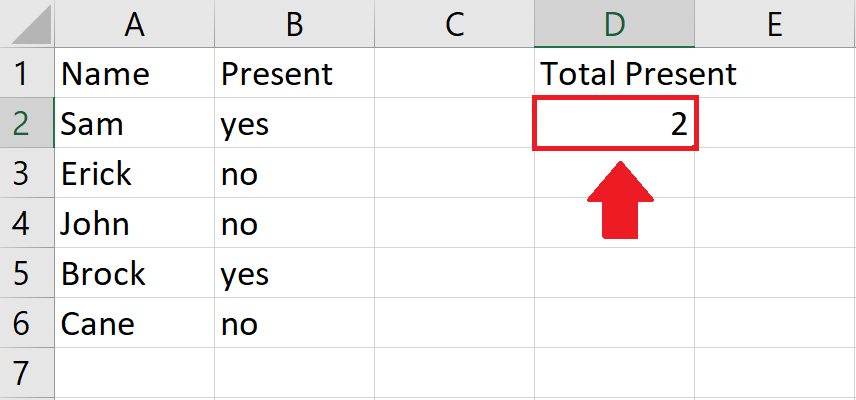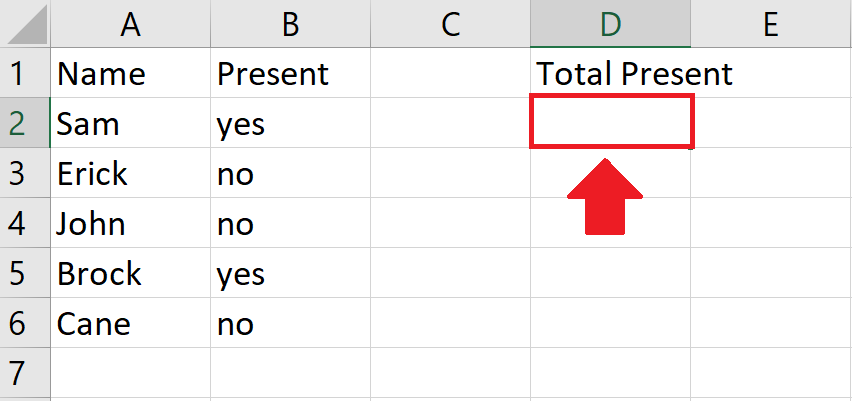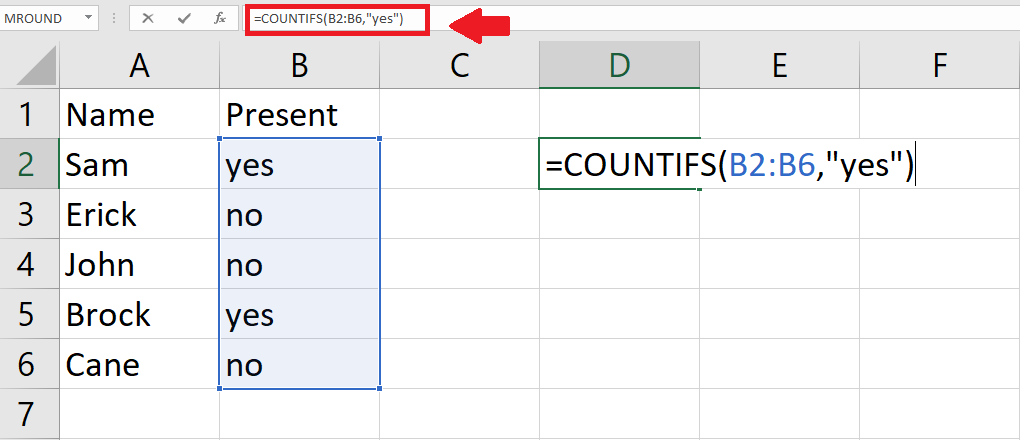# How to count YES in Excel

In this tutorial we will learn how to count repeated words (Yes) in excel. To count the repetition of a “Yes” we use the COUNTFITS function. Following steps guide how to use COUNTIFS functionCOUNTIFS is a function that is used to find how many times a word is repeated in a document. If you have a large database that contains yes or no words repeatedly, it  becomes difficult to find how many times a word is repeated. Here the COUNTIFS function is used to analyze data easily.

### Step 1 – Select a Cell– Select a cell where you want to show how many times “yes” is repeated

### Step 2 – Use COUNTIFS Function– Use COUNTIFS Function From Insert Function option to Calculate the Repetition of “Yes” term
– We’ll use COUNTIFS Function From Insert Function option to Calculate the Repeated term.

Syntax of COUNTIFS function is:
=COUNTIFS(range1, criteria1, [range2], [criteria2],…)
range1 refers to the Range of data from which we want to count the repeated value
criteria1 refers to the address of cell that contains the word for which the count of occurance is required

– COUNTIFS can be used to test multiple conditions at the same time, however, we’ll use it to test only one condition and i.e to search and count the occurrences of word yes in the data range.

### Step 3 – Press Enter key– After completing parameters of Count function press Enter key to get number of repetition in required cell.